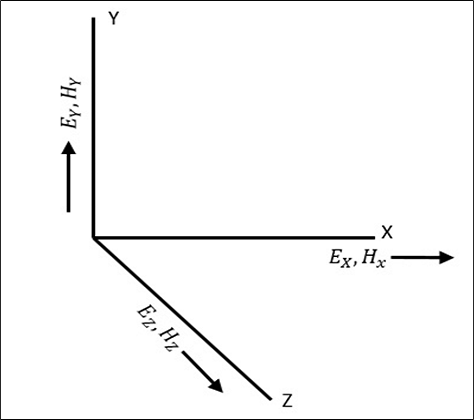# Modes of Propagation

A wave has both electric and magnetic fields. All transverse components of electric and magnetic fields are determined from the axial components of electric and magnetic field, in the z direction. This allows mode formations, such as TE, TM, TEM and Hybrid in microwaves. Let us have a look at the types of modes.

The direction of the electric and the magnetic field components along three mutually perpendicular directions x, y, and z are as shown in the following figure.## Types of Modes

The modes of propagation of microwaves are −

### TEM (Transverse Electromagnetic Wave)

In this mode, both the electric and magnetic fields are purely transverse to the direction of propagation. There are no components in $'Z'$ direction.

$$E_z = 0 \: and \: H_z = 0$$

### TE (Transverse Electric Wave)

In this mode, the electric field is purely transverse to the direction of propagation, whereas the magnetic field is not.

$$E_z = 0 \: and \: H_z \ne 0$$

### TM (Transverse Magnetic Wave)

In this mode, the magnetic field is purely transverse to the direction of propagation, whereas the electric field is not.

$$E_z \ne 0 \: and \: H_z = 0$$

### HE (Hybrid Wave)

In this mode, neither the electric nor the magnetic field is purely transverse to the direction of propagation.

$$E_z \ne 0 \: and \: H_z \ne 0$$

Multi conductor lines normally support TEM mode of propagation, as the theory of transmission lines is applicable to only those system of conductors that have a go and return path, i.e., those which can support a TEM wave.

Waveguides are single conductor lines that allow TE and TM modes but not TEM mode. Open conductor guides support Hybrid waves. The types of transmission lines are discussed in the next chapter.